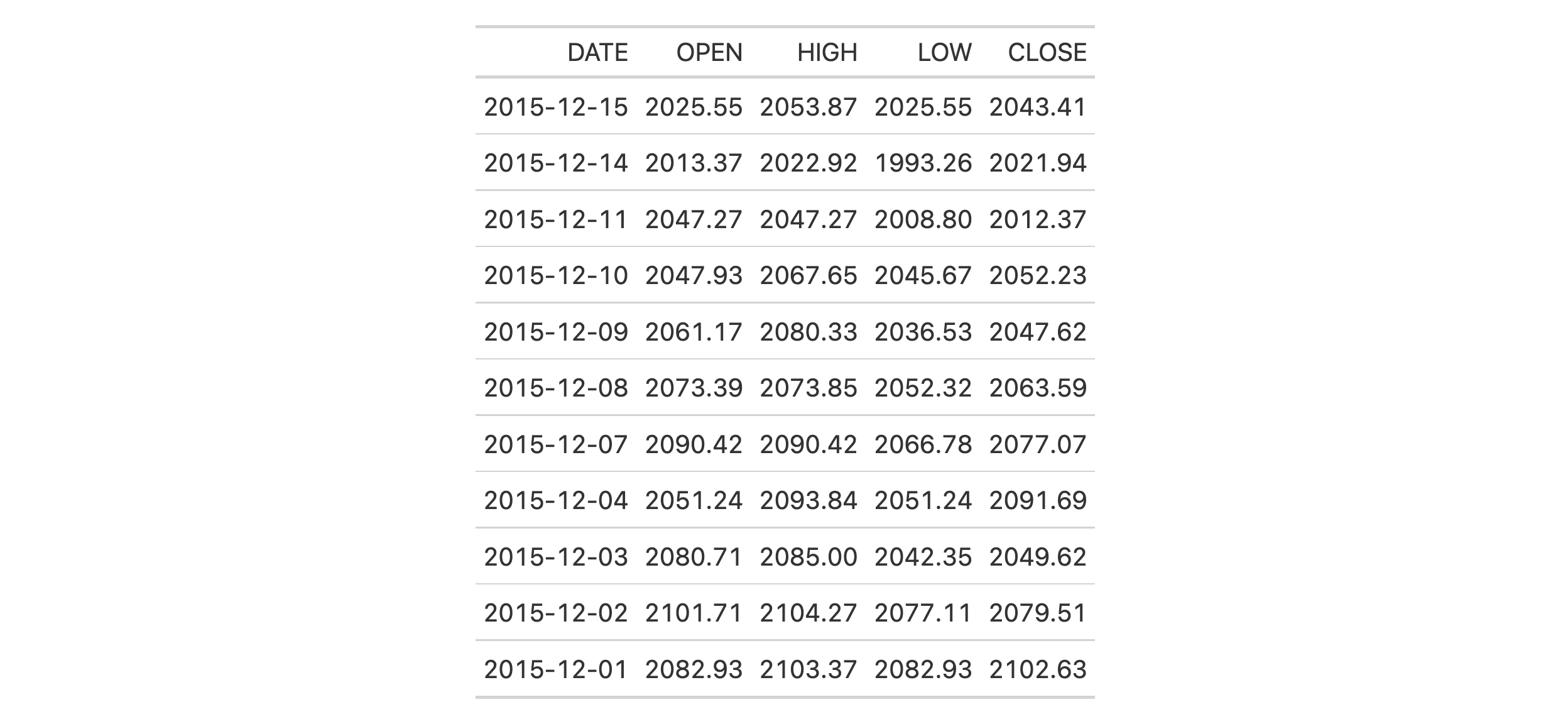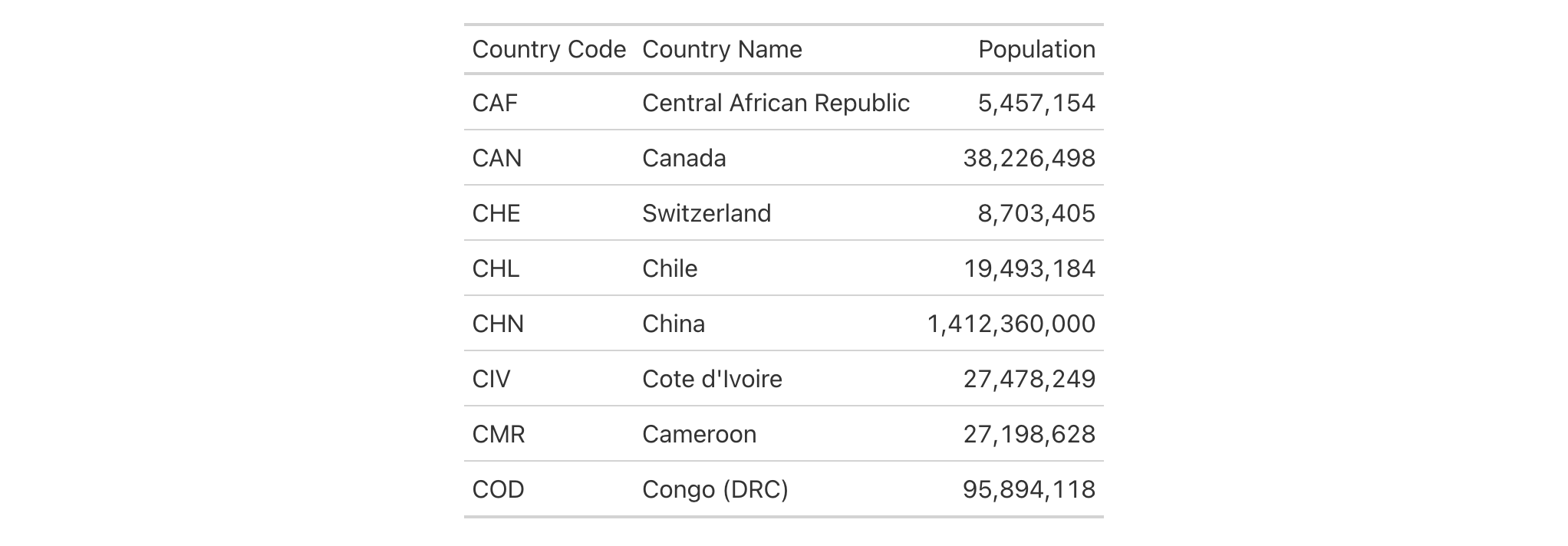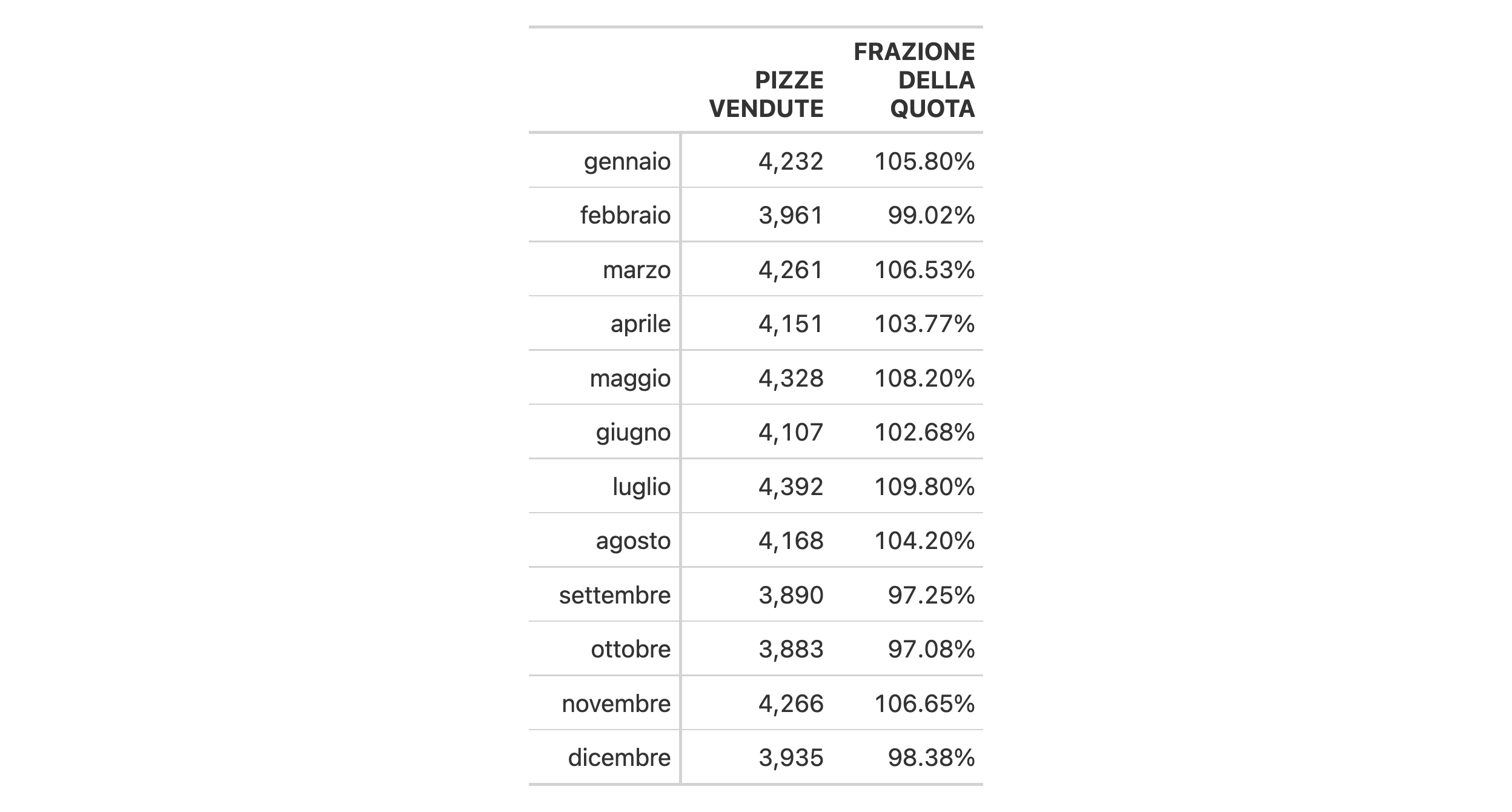# cols_label_with: Relabel columns with a function In gt: Easily Create Presentation-Ready Display Tables

 cols_label_with R Documentation

## Relabel columns with a function

### Description

Column labels can be modified from their default values (the names of the columns from the input table data). When you create a gt table object using `gt()`, column names effectively become the column labels. While this serves as a good first approximation, you may want to make adjustments so that the columns names present better in the gt output table. The `cols_label_with()` function allows for modification of column labels through a supplied function. By default, the function will be invoked on all column labels but this can be limited to a subset via the `columns` argument. With the `fn` argument, we provide either a bare function name, a RHS formula (with `.` representing the vector of column labels), or, an anonymous function (e.g., `function(x) tools::toTitleCase(x)`).

### Usage

``````cols_label_with(data, columns = everything(), fn)
``````

### Arguments

 `data` A table object that is created using the `gt()` function. `columns` The column names to which the function or function call in `fn` should be applied. By default this is set as `everything()` which select every column in the table. `fn` The function or function call to be applied to the column labels. This can take the form of a bare function (e.g., `tools::toTitleCase`), a function call as a RHS formula (e.g., `~ tools::toTitleCase(.)`), or an anonymous function as in `function(x) tools::toTitleCase(x)`.

### Value

An object of class `gt_tbl`.

### A note on column names and column labels

It's important to note that while columns can be freely relabeled, we continue to refer to columns by their original column names. Column names in a tibble or data frame must be unique whereas column labels in gt have no requirement for uniqueness (which is useful for labeling columns as, say, measurement units that may be repeated several times—usually under different spanner column labels). Thus, we can still easily distinguish between columns in other gt function calls (e.g., in all of the `⁠fmt*()⁠` functions) even though we may lose distinguishability in column labels once they have been relabeled.

### Examples

Use `sp500` to create a gt table. We want all the column labels to be entirely capitalized versions of the default labels but, instead of using `cols_label()` and rewriting each label manually in capital letters we can use `cols_label_with()` and instruct it to apply the `toupper()` function to all column labels.

```sp500 |>
dplyr::filter(
date >= "2015-12-01" &
date <= "2015-12-15"
) |>
gt() |>
cols_label_with(fn = toupper)
```Use `countrypops` to create a gt table. To improve the presentation of the table, we are again going to change the default column labels via function calls supplied within `cols_label_with()`. We can, if we prefer, apply multiple types of column label changes in sequence with multiple calls of `cols_label_with()`. Here, we use the `make_clean_names()` functions from the janitor package and follow up with the removal of a numeral with `gsub()`.

```countrypops |>
dplyr::filter(year == 2021) |>
dplyr::filter(grepl("^C", country_code_3)) |>
dplyr::select(-country_code_2, -year) |>
gt() |>
cols_move_to_start(columns = country_code_3) |>
fmt_integer(columns = population) |>
cols_label_with(
fn = ~ janitor::make_clean_names(., case = "title")
) |>
cols_label_with(
fn = ~ gsub("[0-9]", "", .)
)
```We can make a svelte gt table with the `pizzaplace` dataset. There are ways to use one instance of `cols_label_with()` with multiple functions called on the column labels. In the example, we use an anonymous function call (with the `function(x) { ... }` construction) to perform multiple mutations of `x` (the vector of column labels). We can even use the `md()` helper function with that to signal to gt that the column label should be interpreted as Markdown text.

```pizzaplace |>
dplyr::mutate(month = substr(date, 6, 7)) |>
dplyr::group_by(month) |>
dplyr::summarize(pizze_vendute = dplyr::n()) |>
dplyr::ungroup() |>
dplyr::mutate(frazione_della_quota = pizze_vendute / 4000) |>
dplyr::mutate(date = paste0("2015/", month, "/01")) |>
dplyr::select(-month) |>
gt(rowname_col = "date") |>
fmt_date(date, date_style = "month", locale = "it") |>
fmt_percent(columns = frazione_della_quota) |>
fmt_integer(columns = pizze_vendute) |>
cols_width(everything() ~ px(100)) |>
cols_label_with(
fn = function(x) {
janitor::make_clean_names(x, case = "title") |>
toupper() |>
stringr::str_replace_all("^|\$", "**") |>
md()
}
)
```### Function ID

5-5

Other column modification functions: `cols_align_decimal()`, `cols_align()`, `cols_hide()`, `cols_label()`, `cols_merge_n_pct()`, `cols_merge_range()`, `cols_merge_uncert()`, `cols_merge()`, `cols_move_to_end()`, `cols_move_to_start()`, `cols_move()`, `cols_unhide()`, `cols_width()`